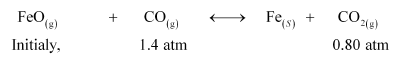# One of the reactions that takes place in producing steel from iron ore is the reduction of ironQuestion:

One of the reactions that takes place in producing steel from iron ore is the reduction of iron (II) oxide by carbon monoxide to give iron metal and CO2.

$\mathrm{FeO}(\mathrm{s})+\mathrm{CO}(\mathrm{g}) \longleftrightarrow \mathrm{Fe}(\mathrm{s})+\mathrm{CO}_{2}(\mathrm{~g}) ; \mathrm{K}_{p}=0.265$ at $1050 \mathrm{~K}$

What are the equilibrium partial pressures of $\mathrm{CO}$ and $\mathrm{CO}_{2}$ at $1050 \mathrm{~K}$ if the initial partial pressures are: $p_{\mathrm{CO}}=1.4 \mathrm{~atm}$ and $p_{\mathrm{CO}_{2}}=0.80$ atm?

Solution:

For the given reaction,$Q_{\mathrm{p}}=\frac{p_{\mathrm{CO}_{2}}}{p_{\mathrm{CO}}}$

$=\frac{0.80}{1.4}$

$=0.571$

It is given that $K_{\mathrm{p}}=0.265$.

Since $Q_{\mathrm{p}}>K_{\mathrm{p}}$, the reaction will proceed in the backward direction.

Therefore, we can say that the pressure of $\mathrm{CO}$ will increase while the pressure of $\mathrm{CO}_{2}$ will decrease.

Now, let the increase in pressure of $\mathrm{CO}=$ decrease in pressure of $\mathrm{CO}_{2}$ be $p$.

Then, we can write,

$K_{\mathrm{P}}=\frac{p_{\mathrm{CO}_{2}}}{p_{\mathrm{CO}}}$

$\Rightarrow 0.265=\frac{0.80-p}{1.4+p}$

$\Rightarrow 0.371+0.265 p=0.80-p$

$\Rightarrow 1.265 p=0.429$

$\Rightarrow p=0.339 \mathrm{~atm}$

Therefore, equilibrium partial of $\mathrm{CO}_{2}, p_{\mathrm{CO}_{2}}=0.80-0.339=0.461 \mathrm{~atm}$.

And, equilibrium partial pressure of $\mathrm{CO}, p_{\mathrm{CO}}=1.4+0.339=1.739 \mathrm{~atm}$.